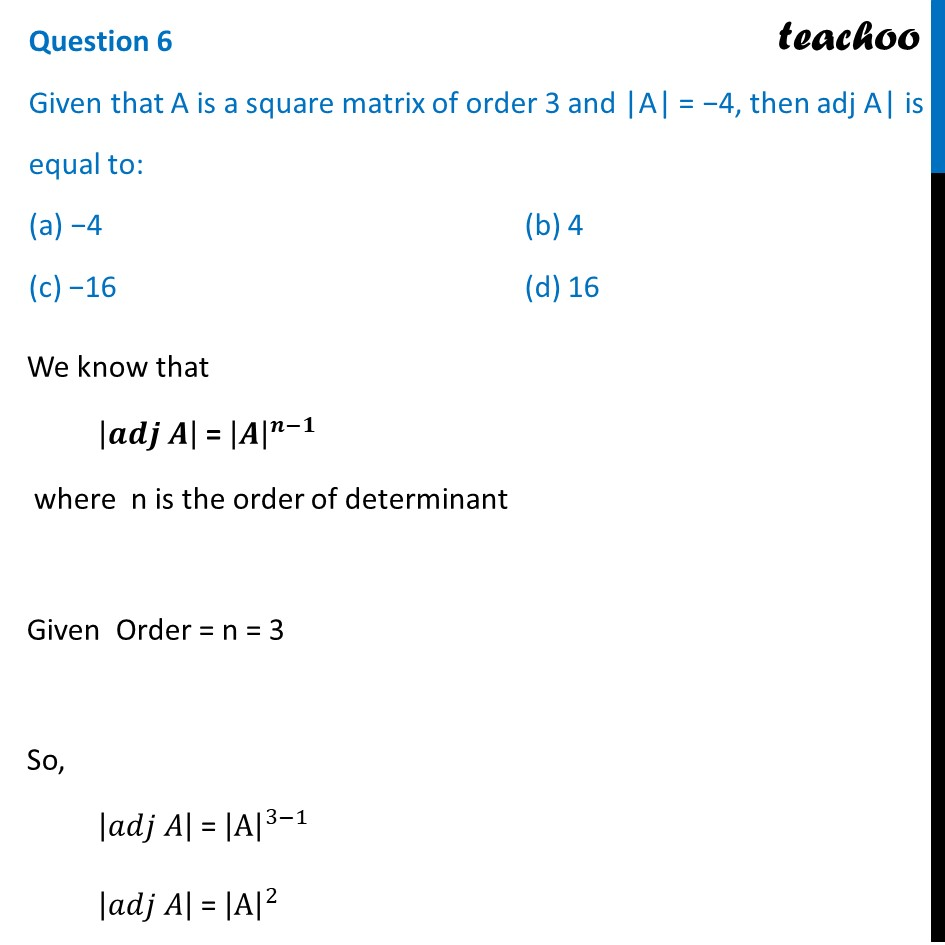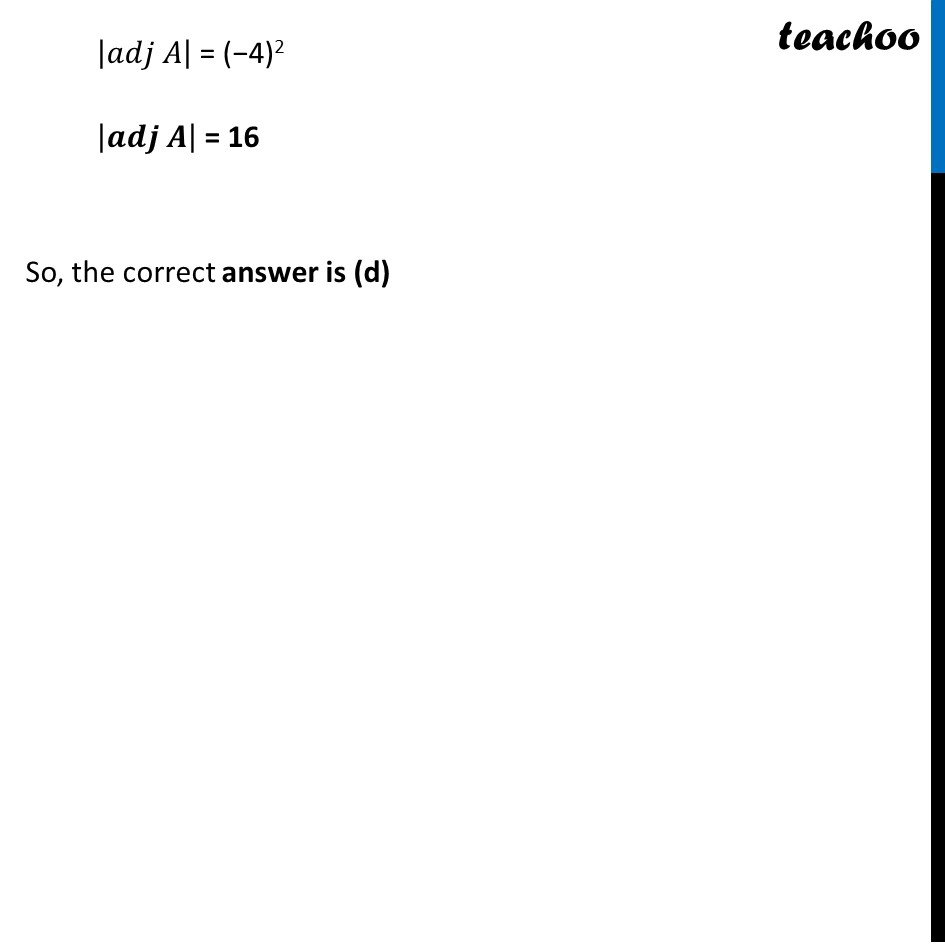CBSE Class 12 Sample Paper for 2022 Boards (MCQ Based - for Term 1)

Class 12
Solutions of Sample Papers and Past Year Papers - for Class 12 Boards

## (a) −4     (b) 4 (c) −16   (d) 16

This question is inspired from Question 5 (Choice 2) - CBSE Class 12 Sample Paper for 2021 BoardsLearn in your speed, with individual attention - Teachoo Maths 1-on-1 Class

### Transcript

Question 6 Given that A is a square matrix of order 3 and |A| = −4, then adj A| is equal to: (a) −4 (b) 4 (c) −16 (d) 16 We know that |𝒂𝒅𝒋 𝑨| = |𝑨|^(𝒏−𝟏) where n is the order of determinant Given Order = n = 3 So, |𝑎𝑑𝑗 𝐴| = |A|^(3−1) |𝑎𝑑𝑗 𝐴| = |A|^2 |𝑎𝑑𝑗 𝐴| = (−4)2 |𝒂𝒅𝒋 𝑨| = 16 So, the correct answer is (d)